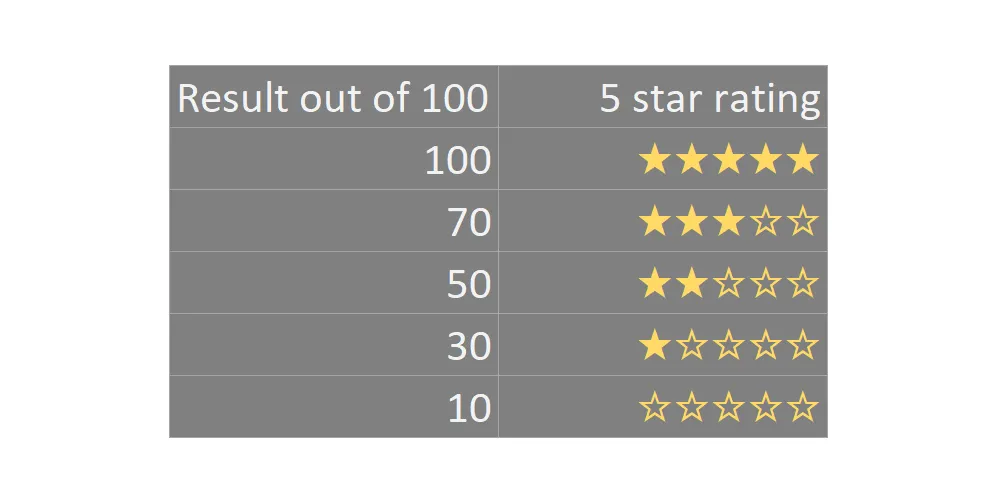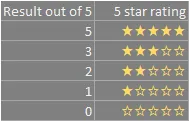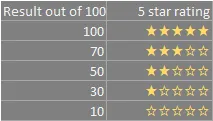# Create star rating in Excel or Power BI using DAX

Here is a simple solution to create a five star rating in Excel using formulas or Power BI using DAX. The result is obtainable using only Excel formulas or DAX capabilities. You can do that with other symbols or emojis too. With the same techniques, it is possible to create a progress bar in Excel or Power BI.

## Star rating in Excel

Let’s get the star symbol in Excel first. There are many ways to get that. You can use Excel built-in symbols, copy symbols from this site and paste them into the formula, or use the function CHAR.

I prefer to copy and paste symbols, and you can see them in the formulas below. In the simplest scenario, if you have a result out of five in column A, you can use two REPT functions and concatenation. The first one will give you full stars, and the second one will add empty stars if there is no maximum.

`=REPT("★", A2) & REPT("☆", 5 - A2)`If you have a result that is not precisely in a five-star rating, you can make additional calculations like this. Here is a situation where the maximum result is 100.

`=REPT("★", INT(A2/(100/5))) & REPT("☆", 5 - INT(A2/(100/5)))`Function INT will round down the result. You can modify that with other functions like the ROUNDUP or the MROUND if there is another principle.

By using the Excel function LET, you can create variables and optimize the solution like this.

```=LET(stars, INT(A2/(100/5)),
REPT("★", stars) & REPT("☆", 5 - stars))```

I prefer to copy and paste symbols, but with the function CHAR, you can get a star like this. In that case, you should use Wingdings font that contains other cool symbols.

`=CHAR(171)`

## Star rating in Power BI using DAX

If you want to create a star rating in Power BI using DAX, you can create a measure for that. You can copy and paste the star symbol or use the function UNICHAR to get that.

Here are both ways.

```Star rating =
VAR max_stars = 5
VAR max_result = 100
VAR full_stars =
INT ( DIVIDE ( SUM ( result[Result out of 100] ), ( max_result / max_stars ) ) )
VAR empty_stars = max_stars - full_stars

RETURN
REPT ( "★", full_stars ) & REPT ( "☆", empty_stars )```

```Star rating with UNICHAR =
VAR max_stars = 5
VAR max_result = 100
VAR full_stars =
INT ( DIVIDE ( SUM ( result[Result out of 100] ), ( max_result / max_stars ) ) )
VAR empty_stars = max_stars - full_stars

RETURN
REPT ( UNICHAR ( 9733 ), full_stars ) & REPT ( UNICHAR ( 9734 ), empty_stars )```

For me, symbols in the code are easy to overlook because I don’t know off the top of my mind the Unicode. Another option is to use a star rating from Power BI quick measures with a slightly different approach to giving a star.

If you want to know how to organize DAX measures, here is a post about that from this blog.

### Rating with half-star in Excel or Power BI with DAX

At this moment, I think it is not possible to use half star character. I only found out about this proposal to incorporate that in Unicode.

The workaround is to use star conditional formatting in Excel and Power BI. It is necessary to create a column for each star, like in this example for Power BI or Excel. I think it is better to live with available star characters and not generate extra columns.